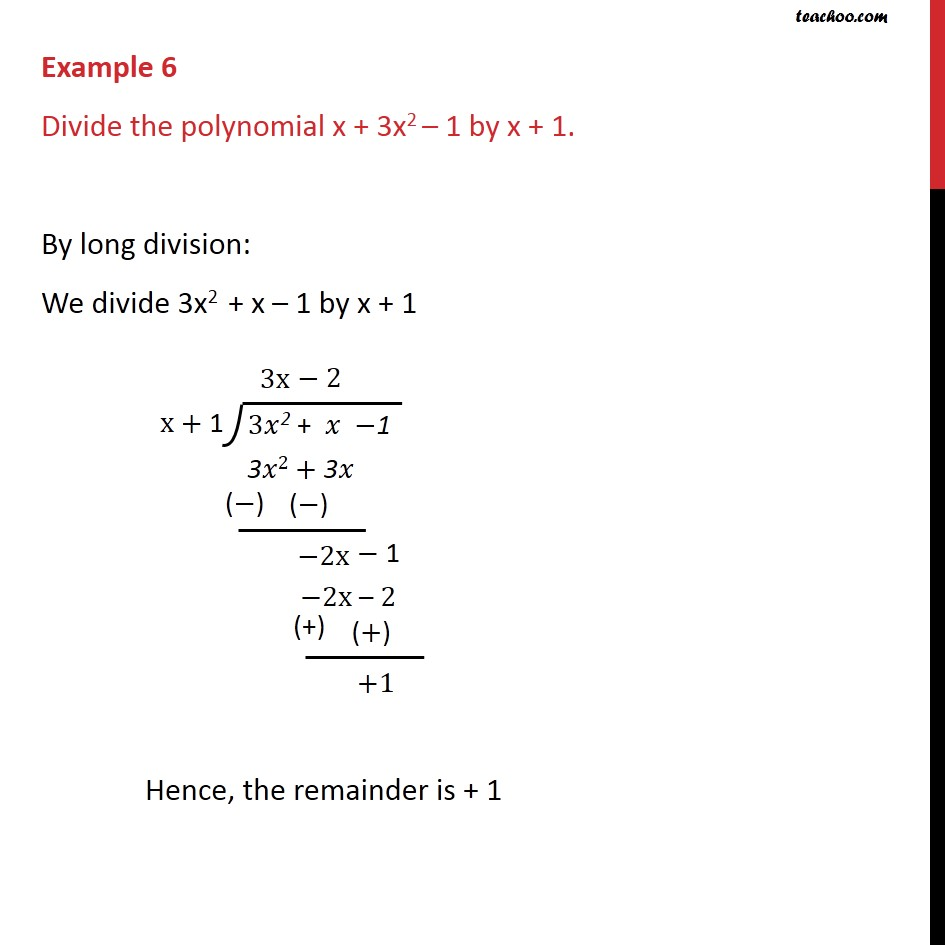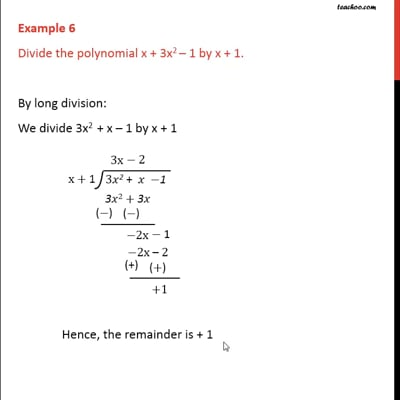Examples

Chapter 2 Class 9 Polynomials
Serial order wiseThis video is only available for Teachoo black users

Introducing your new favourite teacher - Teachoo Black, at only ₹83 per month

### Transcript

Example 6 Divide the polynomial x + 3x2 – 1 by x + 1. By long division: We divide 3x2 + x – 1 by x + 1 We follow these steps 1. We multiply by 3x 2. The remainder will be the difference of 3x2 + x, and 3x2 + 3x i.e. -2x - 1 3. So, we multiply by -2 4. Then, the remainder will be difference of -2x - 1 and -2x - 2 5. Remainder is +1